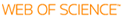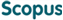### BROWSE

#### Related ResearcherKim, Jingook
Integrated Circuit and Electromagnetic Compatibility Laboratory (IC & EMC Lab)
Research Interests
• Convergence between circuit and EM domains

Inductance Calculations for Plane-Pair Area Fills With Vias in a Power Distribution Network Using a Cavity Model and Partial Inductances

Cited 1 times inCited 4 times inTitle
Inductance Calculations for Plane-Pair Area Fills With Vias in a Power Distribution Network Using a Cavity Model and Partial Inductances
Author
Keywords
Cavity model; decoupling capacitor; equivalent inductance; inductance; parallel plate; partial element equivalent circuit (PEEC); partial inductance; power distribution network (PDN)
Issue Date
2011-08
Publisher
IEEE-INST ELECTRICAL ELECTRONICS ENGINEERS INC
Citation
IEEE TRANSACTIONS ON MICROWAVE THEORY AND TECHNIQUES, v.59, no.8, pp.1909 - 1924
Abstract
Partial inductances are computed herein for the via transitions between parallel planes. A hybrid method proposed for the inductance calculation correlates the definition of the partial inductance and a resonant cavity model. The hybrid method is corroborated by comparison with the partial-element equivalent-circuit and the cavity methods, as well as measurements. The portions of the plane net and via net inductances are quantified, and the contribution of each plane current to the plane net inductance is quantitatively analyzed.
URI
DOI
10.1109/TMTT.2011.2156807
ISSN
0018-9480
Appears in Collections:
EE_Journal Papers
Files in This Item: